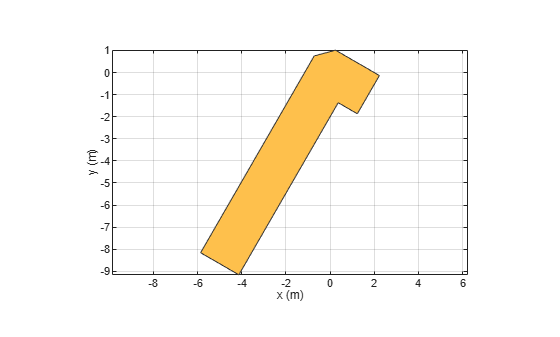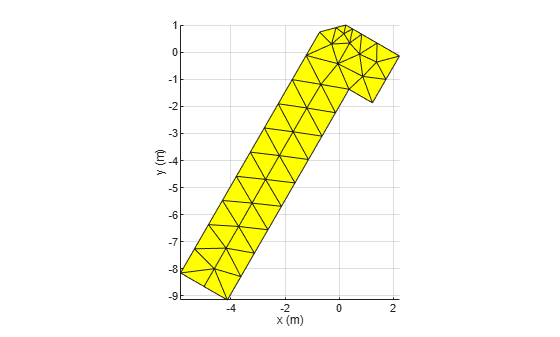# mesh

Change and view mesh properties of metal or dielectric in PCB component

Since R2021b

## Syntax

``mesh(object)``
``mesh(shape)``
``mesh(___,Name,Value)``
``meshdata = mesh(___,Name,Value)``

## Description

````mesh(object)` plots the mesh used to analyze a PCB component.```
````mesh(shape)` plots the mesh for the shapes.```

example

````mesh(___,Name,Value)` changes and plots the mesh structure of a PCB component, using additional options specified by the name-value pairs. You can also determine the number of unknowns from the number of basis functions in the output.```
````meshdata = mesh(___,Name,Value)` returns a mesh structure that specifies the properties used to analyze the PCB component.```

## Examples

collapse all

Create a mitered bend shape of lengths of 10 m and 2 m, width of 2 m, and rotate it about the Z-axis by 60 degrees.

```bend = bendMitered(Length=[10 2],Width=[2 2],MiterDiagonal=1); bend = rotateZ(bend,60)```
```bend = bendMitered with properties: Name: 'myMiteredbend' ReferencePoint: [0 0] Length: [10 2] Width: [2 2] MiterDiagonal: 1 ```
`show(bend)`Mesh the mitered bend shape at a maximum edge length of 1 m.

`mesh(bend,MaxEdgeLength=1)`## Input Arguments

collapse all

PCB component, specified as an object handle. For complete list of PCB components and shapes, see PCB Components Catalog

Shape created using custom elements and shape objects, specified as an object handle. For a complete list of custom shapes, see Custom Geometry and PCB Fabrication.

Example: `c = bendCurved; mesh(c)`

### Name-Value Arguments

Example: `‘MaxEdgeLength’, 0.1`

Specify optional comma-separated pairs of `Name,Value` pair arguments. `Name` is the argument name and `Value` is the corresponding value. `Name` must appear inside single quotes (`''`). You can specify several name and value pair arguments in any order as `Name1, Value1, ..., NameN, ValueN`.

Maximum edge length of triangles in mesh, specified as a positive scalar in meters. All triangles in the mesh have sides less than or equal to the `'MaxEdgeLength'` value.

Data Types: `double`

Smallest edge length of triangles in mesh, specified a positive scalar in meters. All triangles in the mesh have sides greater than or equal to the `'MinEdgeLength'`.

Data Types: `double`

Gradation in the triangle size of the mesh, specified a scalar. The default value of `0.7` states that the growth rate of the mesh is 70 percent. Growth rate values lie between `0` and `1`.

Data Types: `double`

Customize mesh view of a PCB component, specified as a comma-separated pair consisting of `'View'` and `'all'`, `'metal'`,`'dielectric surface'`, or `'dielectric volume'`.

You choose `'dielectric surface'` to view the boundary triangle mesh of the dielectric. You choose `'dielectric volume'` to view the tetrahedral volume mesh of the dielectric.

Data Types: `char`

Option to enable or disable plot interactivity, specified as `'on'` or `'off'`, or as numeric or logical `1(true)` or `0(false)`. Set this argument to `1` or `'on'` to open the plot with the slicer panel, and to slice and view the desired cross section of the plot along the xy-, yz-, and xz- planes. Set this argument to `0` or `'off'` to open the plot without the slicer panel.

Example: `Slicer='on'`

Data Types: `string` | `logical`

## Version History

Introduced in R2021b